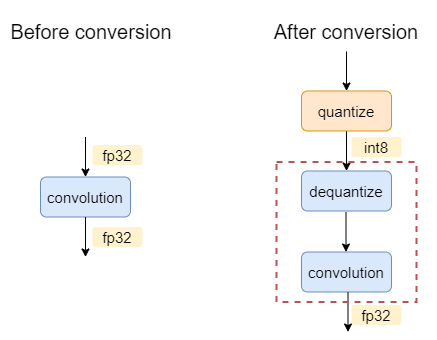# Graph Optimization

Most Deep Learning models could be described as a DAG (directed acyclic graph). Optimizing a deep learning model from a graph perspective is straight forward. Compared to the operator optimization and algorithm optimization, the graph optimization is at a higher level. It covers not only the graph but also the runtime. From the operator perspective, the graph optimization contains the operator fusing and constant folding. From the runtime perspective, the graph optimization contains the operator scheduling, computation resources management, and memory management.

The Intel® Extension for PyTorch* focuses on operator related graph optimizations. The extension also provides some experimental features for the related runtime optimizations. Refer to the runtime extension for more details about runtime optimization.

## Ease-of-use graph optimization API

The graph optimizations of Intel® Extension for PyTorch* are enabled by default. Users can disable it by calling:

```ipex.enable_onednn_fusion(False)
```

### FP32 and BF16 models

```import torch
import torchvision.models as models

# Import the Intel Extension for PyTorch
import intel_extension_for_pytorch as ipex

model = models.resnet50(weights='ResNet50_Weights.DEFAULT')
model.eval()

# Apply some fusions at the front end
model = ipex.optimize(model, dtype=torch.float32)

x = torch.randn(4, 3, 224, 224)
model = torch.jit.trace(model, x, check_trace=False).eval()
# Fold the BatchNormalization and propagate constant
torch.jit.freeze(model)
# Print the graph
print(model.graph_for(x))

print("Execution finished")
```

Compared to the original code, the model launcher needs to add a few lines of code and the extension will automatically accelerate the model. Regarding the RN50, the extension will automatically fuse the Conv + ReLU and Conv + Sum + ReLU as ConvReLU and ConvSumReLU. If you check the output of `graph_for`, you will observe the fused operators.

### INT8 models

```import torch
import torchvision.models as models
import intel_extension_for_pytorch as ipex
from intel_extension_for_pytorch.quantization import prepare, convert

# construct the model
model = models.resnet50(weights='ResNet50_Weights.DEFAULT')
qconfig = ipex.quantization.default_static_qconfig
model.eval()
example_inputs = torch.rand(1, 3, 224, 224)
prepared_model = prepare(model, qconfig, example_inputs=example_inputs, inplace=False)

##### Example Dataloader #####  # noqa F401
import torchvision
DATA = 'datasets/cifar10/'

transform = torchvision.transforms.Compose([
torchvision.transforms.Resize((224, 224)),
torchvision.transforms.ToTensor(),
torchvision.transforms.Normalize((0.5, 0.5, 0.5), (0.5, 0.5, 0.5))
])
train_dataset = torchvision.datasets.CIFAR10(
root=DATA,
train=True,
transform=transform,
)
dataset=train_dataset,
batch_size=128
)

for batch_idx, (d, target) in enumerate(calibration_data_loader):
print(f'calibrated on batch {batch_idx} out of {len(calibration_data_loader)}')
prepared_model(d)
##############################  # noqa F401

convert_model = convert(prepared_model)
traced_model = torch.jit.trace(convert_model, example_inputs)
traced_model = torch.jit.freeze(traced_model)

traced_model.save("quantized_model.pt")

# Deployment
quantized_model = torch.jit.freeze(quantized_model.eval())
images = torch.rand(1, 3, 244, 244)
output = quantized_model(images)

print("Execution finished")
```

## Methodology

### Fusion

#### FP32 and BF16 fusion patterns

• Conv1D/Conv2D/Conv3D/Linear/ConvTranspose2D/ConvTranspose3D + Abs/Clamp/Elu/Exp/GELU/HardTanh/HardSwish/Log/Mish/Sigmoid/Pow/ReLU/Round/Sqrt/Square/Tanh/Leaky_ReLU/SiLU

• Conv1D/Conv2D/Conv3D/Linear/ConvTranspose2D/ConvTranspose3D + Sigmoid + MUL

• Conv1D/Conv2D/Conv3D/Linear + SUM

• Conv1D/Conv2D/Conv3D + SUM + ReLU

• Div + Add + Softmax

• Linear + Linear + Linear

• View + Transpose + Contiguous + View

#### INT8 fusion patterns

The `ipex.quantization.convert(model, conf, inputs)` API will convert an FP32 `torch.nn.Module` to a quantized JIT ScriptModule according to the given quantization recipes.

For example, for a FP32 model of one single convolution, the graph before and after conversion will be:The oneDNN graph backend will select `dequantize` and `convolution` into one partition. During execution, this partition will execute a convolution with int8 as input and fp32 as output.

Here listed all the currently supported int8 patterns in Intel® Extension for PyTorch* using oneDNN graph backend:

1. Conv/Linear/Matmul related fusion patterns

```                                         |
[Quantize]*
|                        |
Dequantize                Dequantize
\                      /
Conv1D/Conv2D/Conv3D/Linear/MatMul
|
[Abs/Elu/GELU/HardTanh/Leaky_ReLU/Sigmoid/
|
[Quantize]*
|
```
```     |              |
Dequantize   Dequantize
\___      ___/
MatMul
\    /
Divide
\   /
|
```
2. Non-Conv/Linear/Matmul related fusion patterns

```           |
Dequantize
|
MaxPool2D
|
Quantize
```
3. INT8-BF16 mixed-precision fusion patterns

```     |              |
Dequantize   Dequantize
|              |
To             To
\___      ___/
MatMul
\      /
[Divide]*
\     /
|
```
```     |              |
Dequantize   Dequantize
|              |
To             To
\___      ___/
MatMul
|
[GeLU]*
|
To
|
Quantize
|
```
```     |              |
Dequantize   Dequantize
|              |
To            To     Dequantize
\___      ___/          |
MatMul              To
\_____        ___/
|
```

### Folding

Stock PyTorch provids constant propagation and BatchNormalization folding. These optimizations are automatically applied to the jit model by invoking `torch.jit.freeze`. Take the Resnet50 as an example:

```import torch
import torchvision.models as models

model = models.resnet50(weights='ResNet50_Weights.DEFAULT')
model.eval()
x = torch.randn(4, 3, 224, 224)

If the model owner does not invoke the `torch.jit.freeze`, the `BatchNormalization` still exists on the graph. Otheriwse, the `BatchNormalization` will be folded on the graph to save the compuation and then improve the performance. Refer to the Constant Folding Wikipedia page for more details.